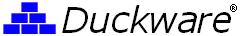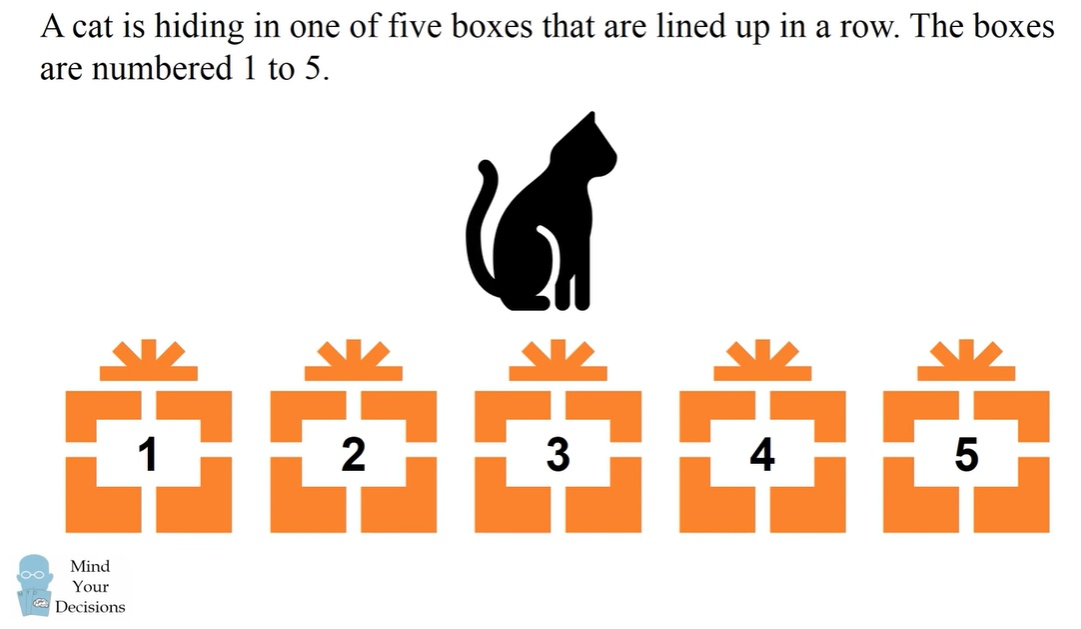You are here: Duckware » Technology » How to solve the "Cat Hiding Puzzle" Contact us
How to solve the "Cat Hiding Puzzle"
(January 18, 2023)A couple of days ago I stumbled across an old puzzle on YouTube, claiming to be a Microsoft interview question. It states:
• A cat is hiding in one of five boxes that are lined up in a row.
• The boxes are numbered 1 to 5.
• Each night the cat hides in an adjacent box, exactly one number away.
• Each morning you can open a single box to try to find the cat.
• Can you win this game of hide and seek? What is your strategy for finding the cat?
The "234" technique: The author (Presh Talwalkar) states that the 'solution' is to guess that the cat is in boxes "2,3,4,2,3,4". This solution works and is guaranteed to find the cat in six (or fewer guesses). And this algorithm will find the in cat -- on average -- in 2.95 guesses.

But, since this problem was also purported to be a Microsoft interview question, is there a faster way (on average) to find the cat?

Because in computer science, a faster 'average speed' algorithm is often a critical deciding factor in which algorithm to use (directly relates to the cost to run that algorithm).

STOP here and try to answer this question yourself before reading on.

The answer is YES you can (on average) find the cat faster, assuming that the cat moves randomly.

Probabilities: An alternative solution can be obtained by guessing at the box with the highest probability of having the cat.
One technique to deal with awkward probability values (being cut in half repeatedly) as the cat can move 50% one way or 50% the other way is to double the denominator and add the numerator to the two possible left/right outcomes boxes. And when the cat is in box 1 or 5, both numerator values are added the adjacent box, as that is the only possible movement. A box that is 'guessed' has its probably value set to zero.
The cat starts by selecting a random box. The probabilities of the cat being in each box are:
 1 1 1 1 1
(out of 5)
and then the cat moves at night (randomly to an adjacent box) and the morning probabilities are:
 1 3 2 3 1
(out of 10)
2: So we guess 'box 2' and find the cat there 3/10 (30%) of the time. But if not, the cat moves at night and the morning probabilities are:
 0 4 3 4 3
(out of 20)
2: So we guess 'box 2' and find the cat there 4/20 (20%) of the time. But if not, the cat moves at night and the morning probabilities are:
 0 3 4 9 4
(out of 40)
4: So we guess 'box 4' and find the cat there 9/40 (22.5%) of the time. But if not, the cat moves at night and the morning probabilities are:
 3 4 3 12 0
(out of 80)
4: So we guess 'box 4' (and find the cat there 12/80 (15%) of the time.

The "2244" technique: I leave it as an exercise for the reader to continue this probability expansion and validate that a very clear pattern emerges -- that explains why "2,2,4,4" is the repeated pattern. This "2,2,4,4" pattern results in the cat being found (on average) in approximately 2.733 guesses.
But because this search method also has the possibility of going on for too long (but statistically, this 'going on for too long' happens incredibly rarely), we can at any time we can cause the guessing to terminate by guessing boxes "2,3,4,2,3,4" (at a very tiny hit on the 2.733 average).
Conclusion: Starting your guessing with "2,2,4,4" (repeatedly for a while) before falling back to guessing "2,3,4" (twice) at some point will result in finding the cat faster -- on average!
• In one guess, "234" = 30%, "2244" = 30%, or equal
• In two guesses, "234" = 45%, "2244" = 50%, a 5% advantage
• In three guesses, "234" = 60%, "2244" = 72.5%, a 12.5% advantage
• In four guesses, "234" = 80%, "2244" = 87.5%, a 7.5% advantage
• In five guesses, "234" = 90%, "2244" = 93.125% a 3.125% advantage
And this behavior explains why the "2244" technique has the statistical advantage over the "234" technique and WILL (on average) find the cat faster.

Why is knowing this important? Because the real cost (\$\$\$) of any algorithm directly depends upon how the algorithm performs, on average (especially when an algorithm is run trillions of times a day)!
For example, some sorting algorithms can have incredibly bad 'worst case' sorting times, but they are used anyway because they perform 'on average' much better than other algorithms.

It is often the case that worse 'worse case' behavior is accepted in exchange for better 'on average' performance!
The lesson learned: The algorithm with the best 'worst case' behavior (finding the cat in six or under guesses) does not mean that that algorithm is the 'best' (or fastest) when it comes to overall (or 'on average') behavior!

Conclusion: In response to the opening question "Can you win this game of hide and seek?" I claim that finding the cat faster (on average) is in fact 'winning' this game.